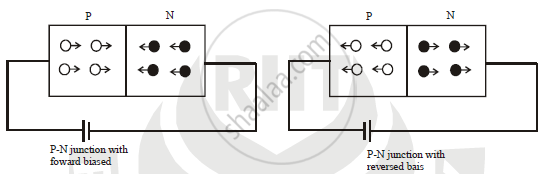# Explain the Working of P-n Junction Diode in Forward and Reverse Biased Mode - Physics

Explain the working of P-N junction diode in forward and reverse biased mode.

#### Solution

If a germanium crystal or silicon crystal is doped during manufacture in such a way that half of it is p-type and other half is n-type, we get p-n junction.(a) Forward biased: A battery is connected across p-n junction diode such that, p-type is connected to positive terminal and n-type is connected to negative terminal, then it is called forward biased. The potential difference applied should be more than 0.3 V for germanium and more than 0.7 V for silicon. Then holes from p-type region and electrons from n-type region moves towards barrier and it decreases width and height of
barrier. Hence an electric current flows through circuit. When applied potential is zero, then current also equal to zero. When P.D. Increases the current is also increases but very slowly. When applied P.D. is more than potential barrier, current in creases rapidly. This voltage is called knee voltage. The forward biased voltage at which the current through diode increases rapidly is called knee voltage

b) Reversed biased : A battery is connected across p-n junction diode such that, p-type is connected to negative terminal and n-type is connected to positive terminal, then it is called reversed biased. The holes from p type region and electrons from n-type region moves away from junction and it increases width and height of barrier. Hence there is no flow of an electric current in ideal case. In actual case current is very small (inμA) . The width of potential barrier increases and diode offer very high resistance. The very small current flows through circuit is called as reverse current. If reversed bias voltage increased then the kinetic energy of electrons increases. At certain reversed bias voltage the K.E. of electrons increases enough and they knock out the electrons from semiconductor atoms\ Therefore current suddenly increase. That certain reversed bias voltage is called as breakdown voltage. At breakdown voltage the current increases suddenly and destroy the junction permanently. The reversed bas voltage at which P-N junction breaks and current suddenly increases is called as breakdown voltage.

Concept: Semiconductor Diode
Is there an error in this question or solution?
2013-2014 (March)

Share